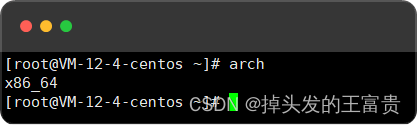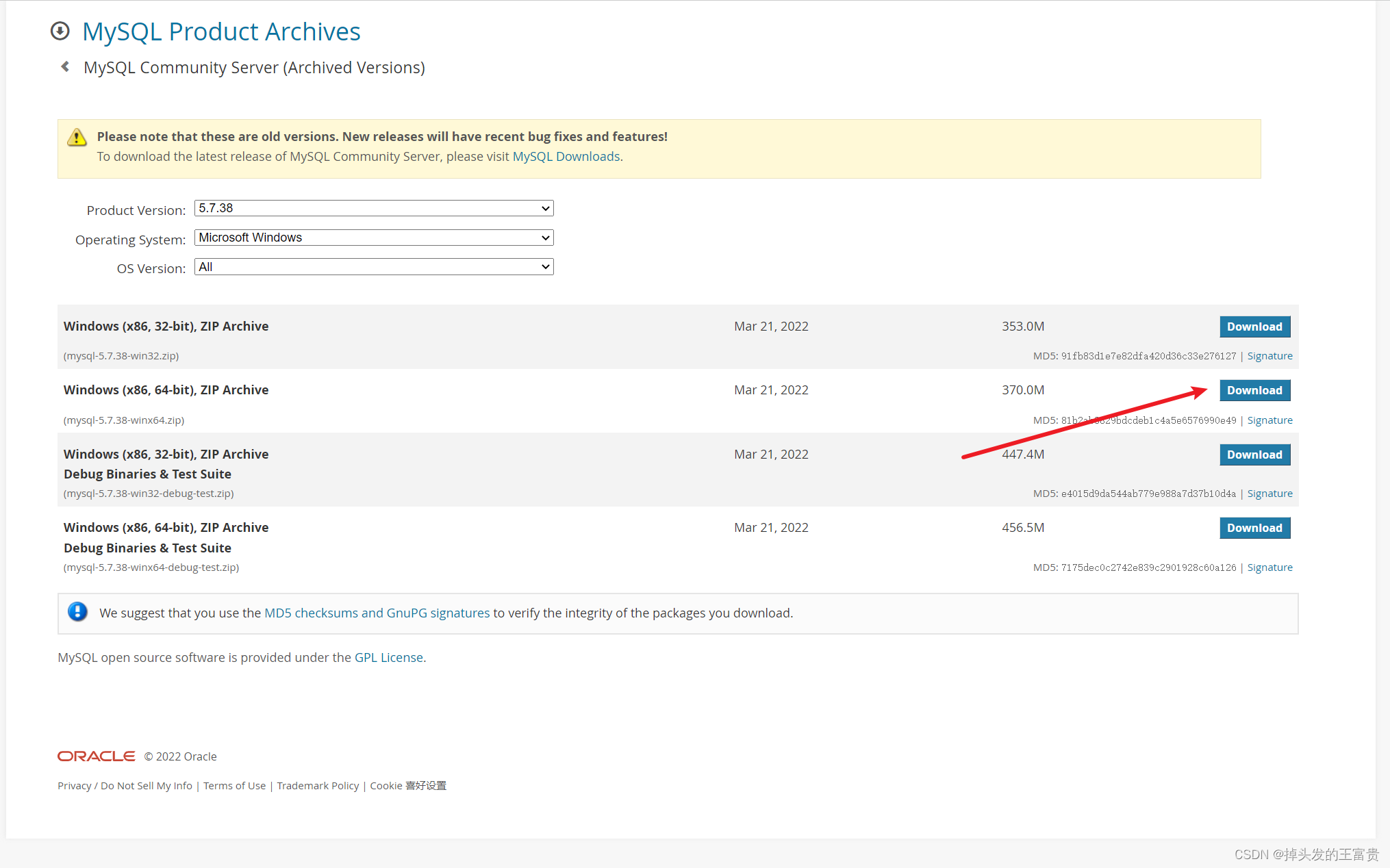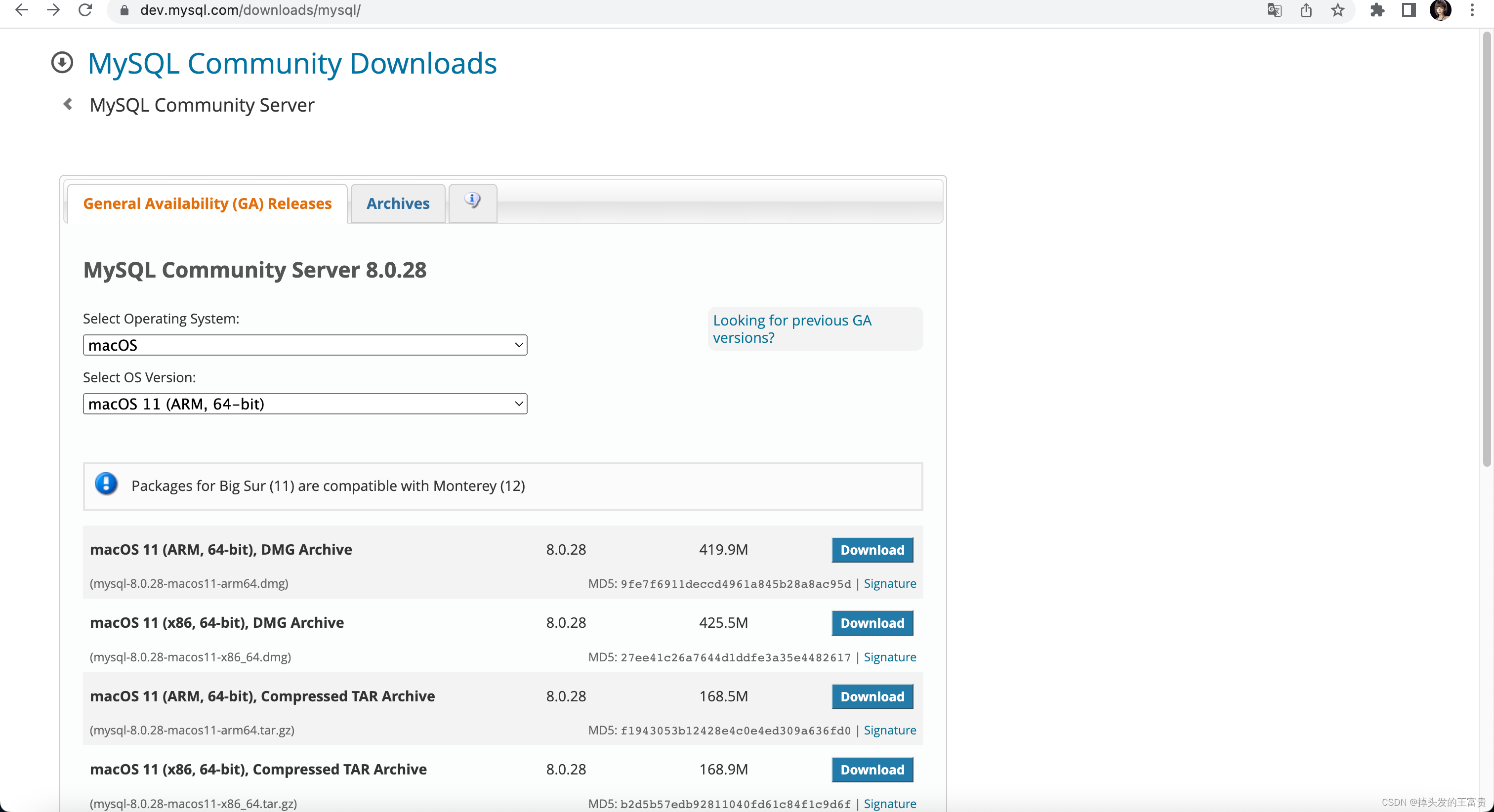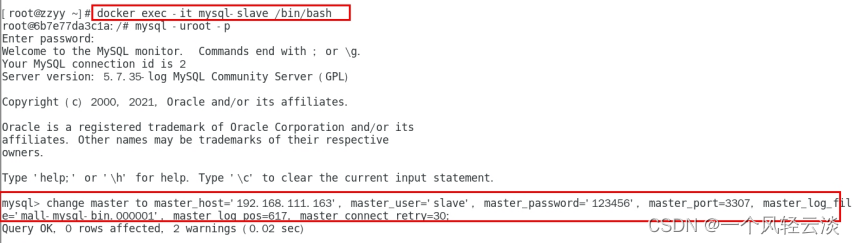# MySQL基础建设之硬盘篇

+关注继续查看

MySQL基础建设之硬盘篇

1.节点统计

2.数据量统计

 某机房 功能业务 data空间使用情况 slow log空间使用情况 bin_log目录使用情况 某同步系统|主库 192G 4.9G 3.2G 某同步系统|从库 281G 43M 3.2G 某api服务|主库 6.2G 300M 3G 某api服务|从库 6.6G 13M 3G 综合业务服务|主库 2.9G 2M 4.8G 综合业务服务|从库 1.4G 92M 3.2G 账号服务 | 主库 4.7G 207M 44G 账号服务 | 从库 43G （*_center 文件占用空间过多） 1M 58M 积分服务 | 主库 1.5G 200M 11G 积分服务 | 从库 2.2G 20M 11G 游戏 | 主库 1.4G 1.8G 753M 游戏 | 从库 1.4G 115M 776M 微博业务   | 主库 2.0G 2.5G 674M 微博业务  | 从库 5.5G  (ibdata2占略大) 8G 661M

3.硬件选型

本文来自 http://yijiu.blog.51cto.com 盗贴 可耻

1.压测工具选择

fio -name iops -rw=write -bs=4k -runtime=60 -iodepth 32 -filename /dev/sdb1 -ioengine libaio -direct=1

fio -name iops -rw=randwrite -bs=4k -runtime=60 -iodepth 32 -filename /dev/sda1 -ioengine libaio -direct=1

fio -name iops -rw=randread -bs=4k -runtime=60 -iodepth 32 -filename /dev/sda1 -ioengine libaio -direct=1

本文来自 http://yi  jiu.bl og.51ct o.com 盗 贴 可耻

[root@localhost io_test]# fio -filename=/io_test/test -iodepth=64 -ioengine=libaio  -direct=1 -rw=randwrite -bs=4k -size=500G -numjobs=64 -runtime=20 -group_reporting -name=test-rand-writell

test-rand-writell: (g=0): rw=randwrite, bs=4K-4K/4K-4K/4K-4K, ioengine=libaio, iodepth=64

...

fio-2.1.10

Starting 64 processes

test-rand-writell: Laying out IO file(s) (1 file(s) / 512000MB)

Jobs: 30 (f=30): [_wwww_w__www______ww___ww___ww_w__ww__ww_w_wwww_w_w_w______w__ww] [3.3% done] [0KB/70497KB/0KB /s] [0/17.7K/0 iops] [eta 11m:20s]

test-rand-writell: (groupid=0, jobs=64): err= 0: pid=4305: Wed Apr  8 00:49:59 2015

write: io=1452.2MB, bw=74239KB/s, iops=18559, runt= 20028msec

slat (usec): min=15, max=80419, avg=3437.87, stdev=8530.70

clat (msec): min=1, max=747, avg=216.14, stdev=107.04

lat (msec): min=1, max=755, avg=219.57, stdev=108.24

clat percentiles (msec):

|  1.00th=[    6],  5.00th=[   30], 10.00th=[   69], 20.00th=[  126],

| 30.00th=[  161], 40.00th=[  190], 50.00th=[  217], 60.00th=[  243],

| 70.00th=[  269], 80.00th=[  306], 90.00th=[  355], 95.00th=[  396],

| 99.00th=[  474], 99.50th=[  506], 99.90th=[  578], 99.95th=[  603],

| 99.99th=[  693]

bw (KB  /s): min=  115, max=38223, per=1.54%, avg=1141.21, stdev=1116.54

lat (msec) : 2=0.55%, 4=0.11%, 10=1.68%, 20=1.63%, 50=3.58%

lat (msec) : 100=7.34%, 250=47.92%, 500=36.62%, 750=0.58%

cpu          : usr=0.24%, sys=1.88%, ctx=424437, majf=0, minf=1842

IO depths    : 1=0.1%, 2=0.1%, 4=0.1%, 8=0.1%, 16=0.3%, 32=0.6%, >=64=98.9%

submit    : 0=0.0%, 4=100.0%, 8=0.0%, 16=0.0%, 32=0.0%, 64=0.0%, >=64=0.0%

complete  : 0=0.0%, 4=100.0%, 8=0.0%, 16=0.0%, 32=0.0%, 64=0.1%, >=64=0.0%

issued    : total=r=0/w=371717/d=0, short=r=0/w=0/d=0

latency   : target=0, window=0, percentile=100.00%, depth=64

Run status group 0 (all jobs):

WRITE: io=1452.2MB, aggrb=74239KB/s, minb=74239KB/s, maxb=74239KB/s, mint=20028msec, maxt=20028msec

sda: ios=0/371765, merge=0/13948, ticks=0/2776215, in_queue=2776513, util=99.24%

IOPS只有1.8W ，那么需要考虑的为啥只有1.8W 那么继续往下看

本 文来 自 ht tp://y ijiu.bl og.51 cto .com 盗 贴可耻

2.压测工具命令各参数

fio -filename=/io_test/test -iodepth=64 -ioengine=libaio  -direct=1 -rw=randwrite -bs=4k -size=500G -numjobs=64 -runtime=20 -group_reporting

Filename                测试文件名称，通常选择需要测试的盘的data目录。

iodepth                 IO深度

-ioengine=libaio        使用libaio模式

direct=1                测试过程绕过自身缓存

bs=4k                   单次io的块文件大小为4k

numjobs=64              本次的测试线程为64

1.缓存

2.IO深度和并发

#接下来可以试着做一个 4 并发 和 一个 8并发的测试来查看结果，可以自行测试，不再放结果了

direct如果是影响 CPU 的话，那么应该是数字越小，CPU消耗越大。因为数字越小，CPU 要批量往硬盘发数据的次数就是越多，但是数字越大，积压的内存理论上应该越多

http://www.edgememory.com/ssds.aspx

RAID阵列卡的产品介绍

http://www.dell.com/learn/us/en/04/campaigns/dell-raid-controllers?c=us&l=en&s=bsd&cs=04&redirect=1

http://blog.xbyte.com/author/lyndsieezell/page/2/

For 2 and 4 disk arrays, performance for 100% sequential I/O was improved by utilizing FastPath.

1、参考其他人的压测经验

本 文来 自 htt p:/ /yiji  u.blog.51cto.com 盗贴 可耻

RAID10的压测结果如下：

6块SSD做的RAID10可能会导致性能的下降，建议使用较少的磁盘数量比数量多的更佳

RAID 5 的压测结果

本文来 自 h ttp :/ /yijiu.blog .51c to.com 盗 贴~可耻

http://www.cnblogs.com/ylqmf/archive/2013/02/28/2936895.html

512kb压测对比

1.FastPATH的作用本文来 自 ht~t p://yi~ji~u.bl~og.51~cto.co~m ~盗贴 可耻

2.开启FastPATH

FastPATH 默认为开启状态；主要作用为直接写入缓存和不进行预读操作

Fastpath I/O (H710P and H810 only)

• Fastpath I/O is a method of increasing Input/Output Operations per Second (IOPS) by using the 2nd core of the PERC (Gemini core) to directly execute I/O and completely bypass caching.

• Fastpath I/O is most beneficial for SSD disks.

• Fastpath I/O is not available on H710 controller, only available on H710P and H810 controllers.

• Fastpath I/O is automatically available on eligible VDs.

Fastpath eligible VDs should have the following properties:

•

• Write-Through

• No Background Operation (e.g. Background Initialization, Patrol Read or Consistency Check ) in progress.

Write-Through          #设置为直接写

本文来自 http://y ~iji~u.bl  og.51cto.com 盗贴可耻

3.回写、直写和预读、非预读

本文来 自 ht~t p://yi~ji~u.bl~og.51~cto.co~m ~盗贴 可耻本文来   自  ht~t p://yi~ji~u. bl~og .51~cto.co~m ~盗贴 可耻

 压测结果统计 读/写 IOPS 随机写 5200 顺序写 18512 随机读 68000

1.FastPATH特性及生效范围2.选型mysql 硬盘满了 问题
mysql 硬盘满了，导致问题  可以执行 读操作，不能执行写操作，  使用linux  命令 df  -h 查看空间使用率                               捐助开发者 在兴趣的驱动下,写一个免费的东西，有欣喜，也还有汗水，希望你喜欢我的作品，同时也能支持一下。
586 0MYSQL的硬盘IO过高引起的CPU过高判断
6722 0mysql服务器硬盘损坏后的数据恢复

1427 0CentOS mysql硬盘满了挂载阿里云硬盘

791 0Linux安装Mysql（图文解说详细版，安装包tar包版）
Linux安装Mysql（图文解说详细版，安装包tar包版）
0 0Windows安装Mysql（图文解说详细版，无msi版本）
Windows安装Mysql（图文解说详细版，无msi版本）
0 0Mac安装Mysql（图文解说详细版）
Mac安装Mysql（图文解说详细版）
0 00 0【云原生】docker安装mysql实现主从复制
【云原生】docker安装mysql实现主从复制
0 0CentOS7 下安装MySQL
CentOS7 下安装MySQL
0 0

MySQL实战进阶5407

Java Spring Boot 2.6.0开发实战-1024程序员节创造营公益课859513667

MySQL企业常见架构与调优经验分享122457443722765